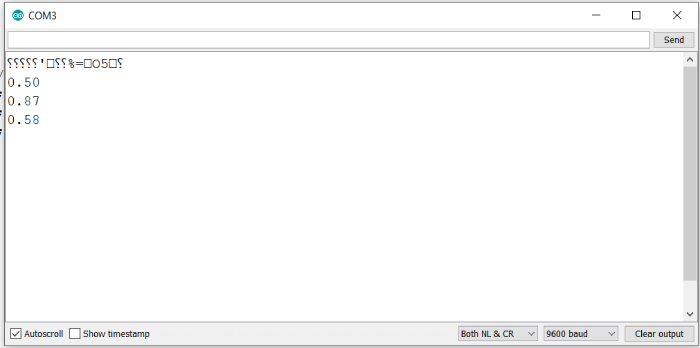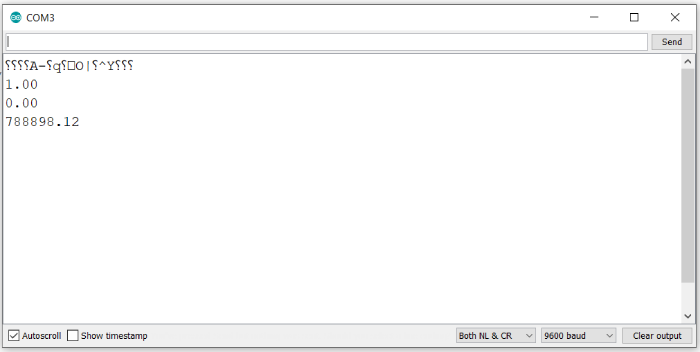# Trigonometric expressions in Arduino

Arduino provides 3 basic trigonometric functions: sin(), cos() and tan(). All other trigonometric expressions can be derived from these three functions.

All the three functions take in angle in radians (type float) as the input. They return a double.

For sin() and cos(), the value is between -1 and 1. The value for tan() has no such bounds.

## Example

The example code below illustrates the use of these functions −

void setup() {
// put your setup code here, to run once:
Serial.begin(9600);
Serial.println();
float pi = 3.14159;
float angle_deg = 30;
}

void loop() {
// put your main code here, to run repeatedly:
}

## Output

The Serial Monitor output is −sin(30°) =1/2, cos(30°) = sqrt(3)/2 = 0.86602, tan(30°) = 1/sqrt(3) = 0.57735. Thus, the values returned are correct.

It will be interesting to see the values printed for 90 degrees, because, tan(90°) is equal to infinity. Let’s try. The Serial Monitor output for the above code, with 30 replaced with 90 is shown below −As you can see, it printed 7,88,898.12 for tan(90 degrees), which is a very high number. Also note that our pi was defined only till an accuracy of 5 decimal places. So the input to the function was not exactly 90 degrees, but a value very close to it.